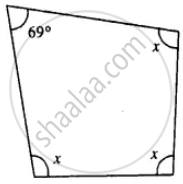# Three Angles of a Quadrilateral Are Equal. If the Fourth Angle is 69°; Find the Measure of Equal Angles. - Mathematics

Sum

Three angles of a quadrilateral are equal. If the fourth angle is 69°; find the measure of equal angles.

#### Solution

Let each equal angle be x°

x + x + x + 69° = 360°3x = 360°- 69

3x = 291

x = 97°

Each, equal angle = 97°

Is there an error in this question or solution?

#### APPEARS IN

Selina Concise Mathematics Class 8 ICSE
Chapter 16 Understanding Shapes
Exercise 16 (C) | Q 9 | Page 187
Selina Class 6 Mathematics